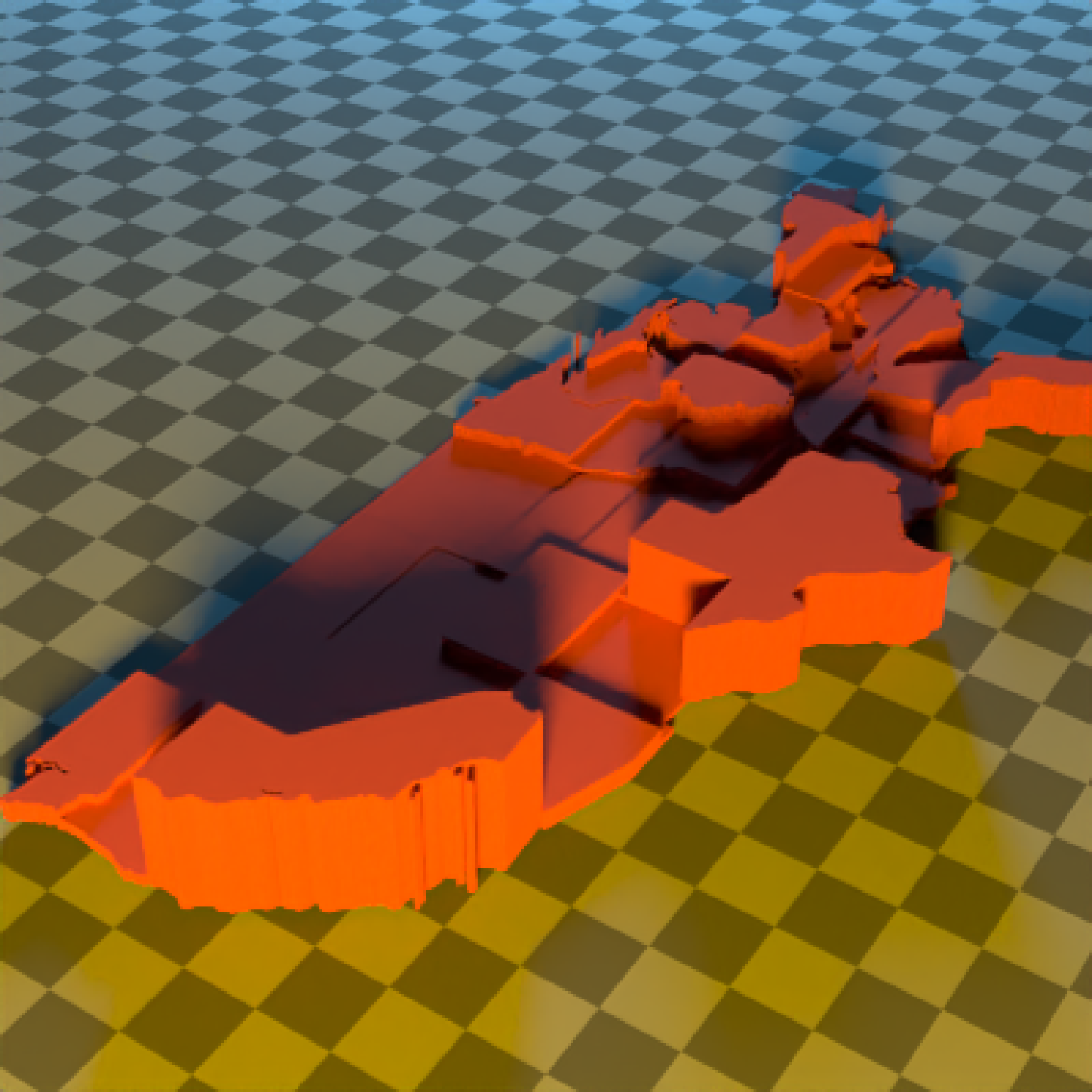Extruded Polygon Object

extruded_polygon(
polygon = NULL,
x = 0,
y = 0,
z = 0,
plane = "xz",
top = 1,
bottom = 0,
holes = NULL,
angle = c(0, 0, 0),
order_rotation = c(1, 2, 3),
material = diffuse(),
center = FALSE,
flip_horizontal = FALSE,
flip_vertical = FALSE,
data_column_top = NULL,
data_column_bottom = NULL,
scale_data = 1,
scale = c(1, 1, 1)
)

## Arguments

polygon

sf object, "SpatialPolygon" sp object, or xy coordinates of polygon represented in a way that can be processed by xy.coords(). If xy-coordinate based polygons are open, they will be closed by adding an edge from the last point to the first. If the sf object contains MULTIPOLYGONZ data, it will flattened.

x

Default 0. x-coordinate to offset the extruded model.

y

Default 0. y-coordinate to offset the extruded model.

z

Default 0. z-coordinate to offset the extruded model.

plane

Default xz. The plane the polygon is drawn in. All possibile orientations are xz, zx, xy, yx, yz, and zy.

top

Default 1. Extruded top distance. If this equals bottom, the polygon will not be extruded and just the one side will be rendered.

bottom

Default 0. Extruded bottom distance. If this equals top, the polygon will not be extruded and just the one side will be rendered.

holes

Default 0. If passing in a polygon directly, this specifies which index represents the holes in the polygon. See the earcut function in the decido package for more information.

angle

Default c(0, 0, 0). Angle of rotation around the x, y, and z axes, applied in the order specified in order_rotation.

order_rotation

Default c(1, 2, 3). The order to apply the rotations, referring to "x", "y", and "z".

material

Default diffuse.The material, called from one of the material functions diffuse, metal, or dielectric.

center

Default FALSE. Whether to center the polygon at the origin.

flip_horizontal

Default FALSE. Flip polygon horizontally in the plane defined by plane.

flip_vertical

Default FALSE. Flip polygon vertically in the plane defined by plane.

data_column_top

Default NULL. A string indicating the column in the sf object to use to specify the top of the extruded polygon.

data_column_bottom

Default NULL. A string indicating the column in the sf object to use to specify the bottom of the extruded polygon.

scale_data

Default 1. If specifying data_column_top or data_column_bottom, how much to scale that value when rendering.

scale

Default c(1, 1, 1). Scale transformation in the x, y, and z directions. If this is a single value, number, the object will be scaled uniformly. Note: emissive objects may not currently function correctly when scaled.

## Value

Multiple row tibble describing the extruded polygon in the scene.

## Examples

#Manually create a polygon object, here a star:

if(run_documentation()) {
angles = seq(0,360,by=36)
xx = rev(c(rep(c(1,0.5),5),1) * sinpi(angles/180))
yy = rev(c(rep(c(1,0.5),5),1) * cospi(angles/180))
star_polygon = data.frame(x=xx,y=yy)
}

if(run_documentation()) {
generate_ground(depth=0,
material = diffuse(color="grey50",checkercolor="grey20")) %>%
material=diffuse(color="red",sigma=90))) %>%
render_scene(parallel=TRUE,lookfrom = c(0,2,3),samples=128,lookat=c(0,0.5,0),fov=60)
}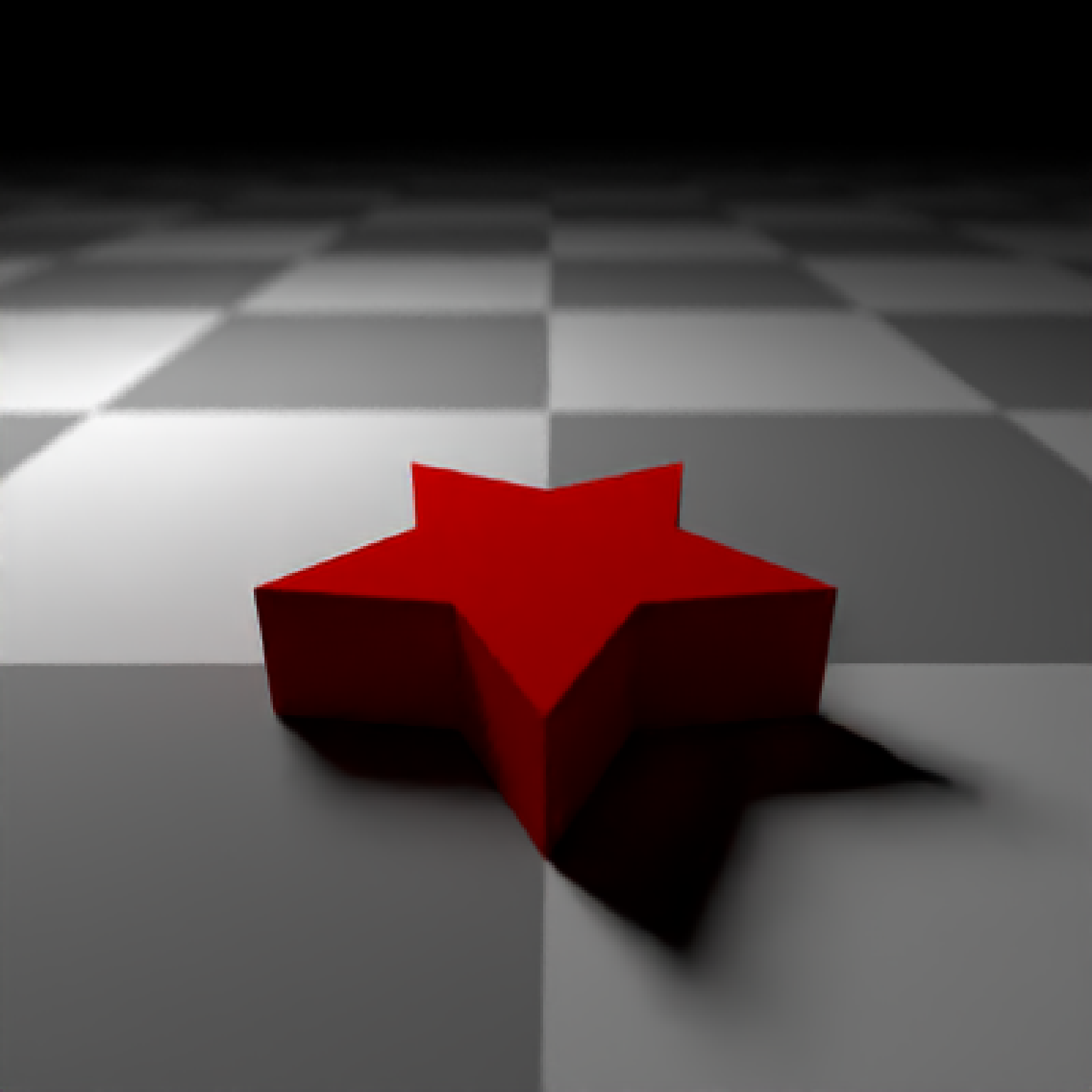#Now, let's add a hole to the center of the polygon. We'll make the polygon
#hollow by shrinking it, combining it with the normal size polygon,
#and specify with the holes argument that everything after nrow(star_polygon)
#in the following should be used to draw a hole:

if(run_documentation()) {
hollow_star = rbind(star_polygon,0.8*star_polygon)
}

if(run_documentation()) {
generate_ground(depth=-0.01,
material = diffuse(color="grey50",checkercolor="grey20")) %>%
add_object(extruded_polygon(hollow_star,top=0.25,bottom=0, holes = nrow(star_polygon) + 1,
material=diffuse(color="red",sigma=90))) %>%
render_scene(parallel=TRUE,lookfrom = c(0,2,4),samples=128,lookat=c(0,0,0),fov=30)
}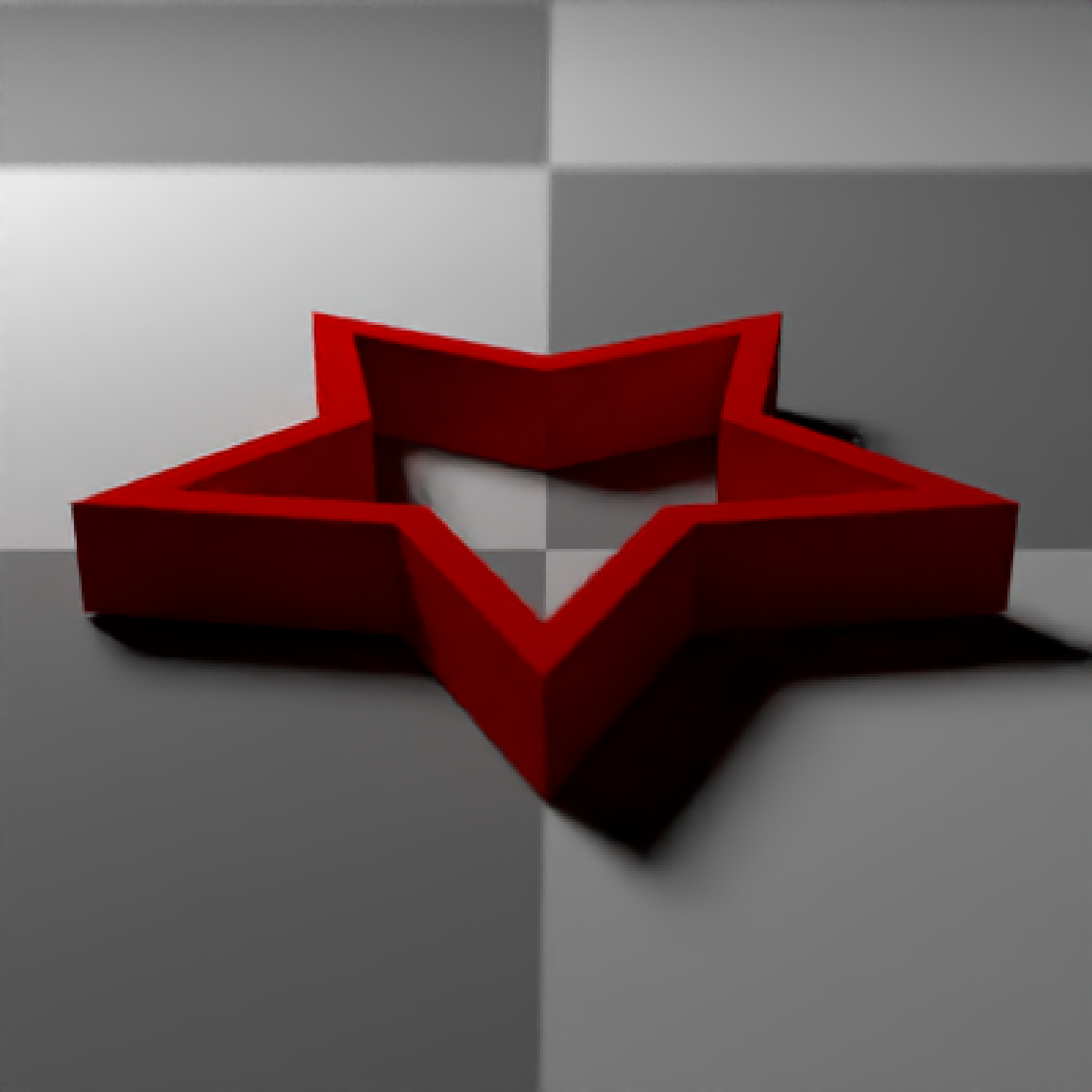# Render one in the y-x plane as well by changing the plane argument,
# as well as offset it slightly.
if(run_documentation()) {
generate_ground(depth=-0.01,
material = diffuse(color="grey50",checkercolor="grey20")) %>%
material=diffuse(color="red",sigma=90))) %>%
holes = nrow(star_polygon) + 1, plane = "yx",
material=diffuse(color="green",sigma=90))) %>%
render_scene(parallel=TRUE,lookfrom = c(0,2,4),samples=128,lookat=c(0,0.9,0),fov=40)
}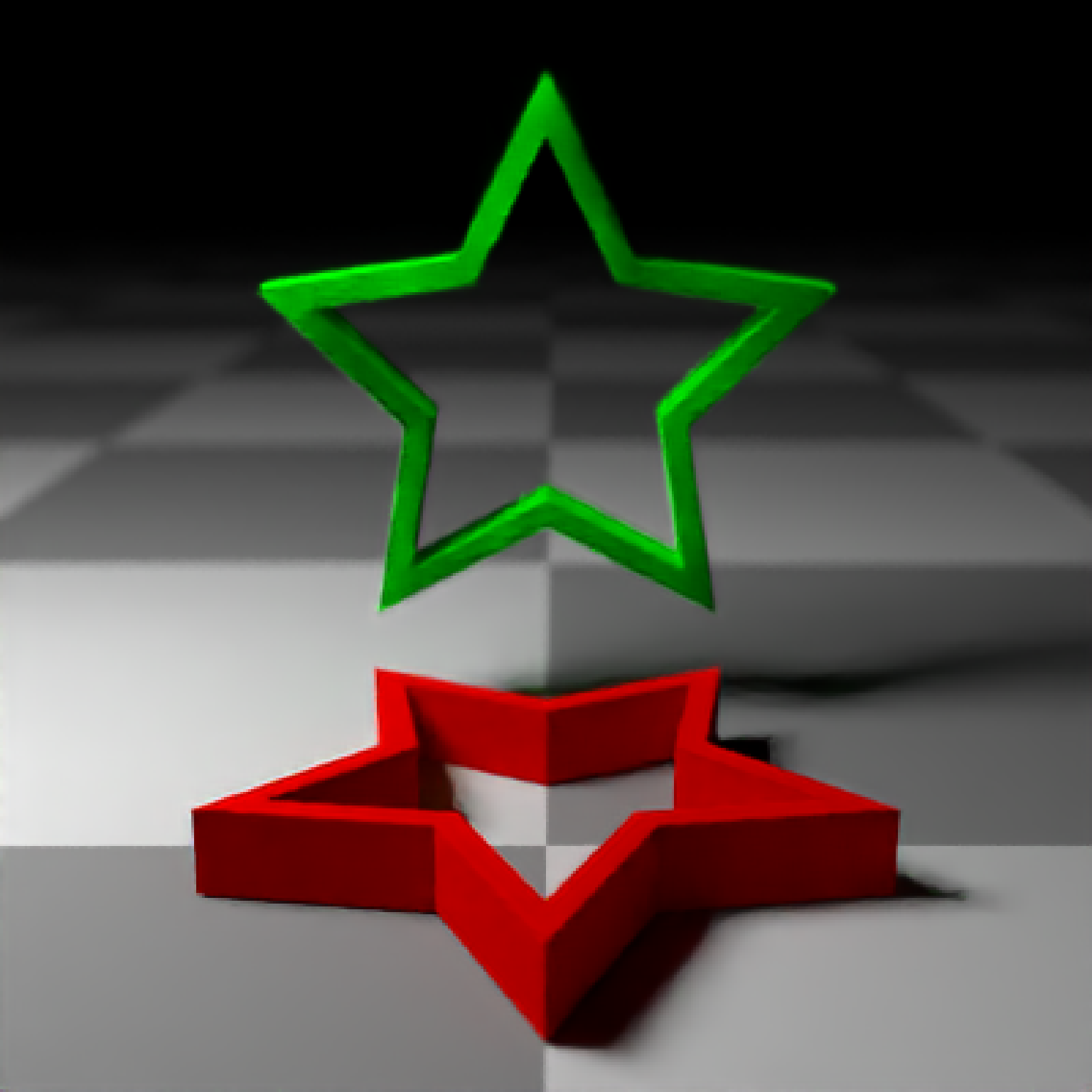# Now add the zy plane:
if(run_documentation()) {
generate_ground(depth=-0.01,
material = diffuse(color="grey50",checkercolor="grey20")) %>%
add_object(extruded_polygon(hollow_star,top=0.25,bottom=0, holes = nrow(star_polygon) + 1,
material=diffuse(color="red",sigma=90))) %>%
holes = nrow(star_polygon) + 1, plane = "yx",
material=diffuse(color="green",sigma=90))) %>%
holes = nrow(star_polygon) + 1, plane = "zy",
material=diffuse(color="blue",sigma=90))) %>%
render_scene(parallel=TRUE,lookfrom = c(-4,2,4),samples=128,lookat=c(0,0.9,0),fov=40)
}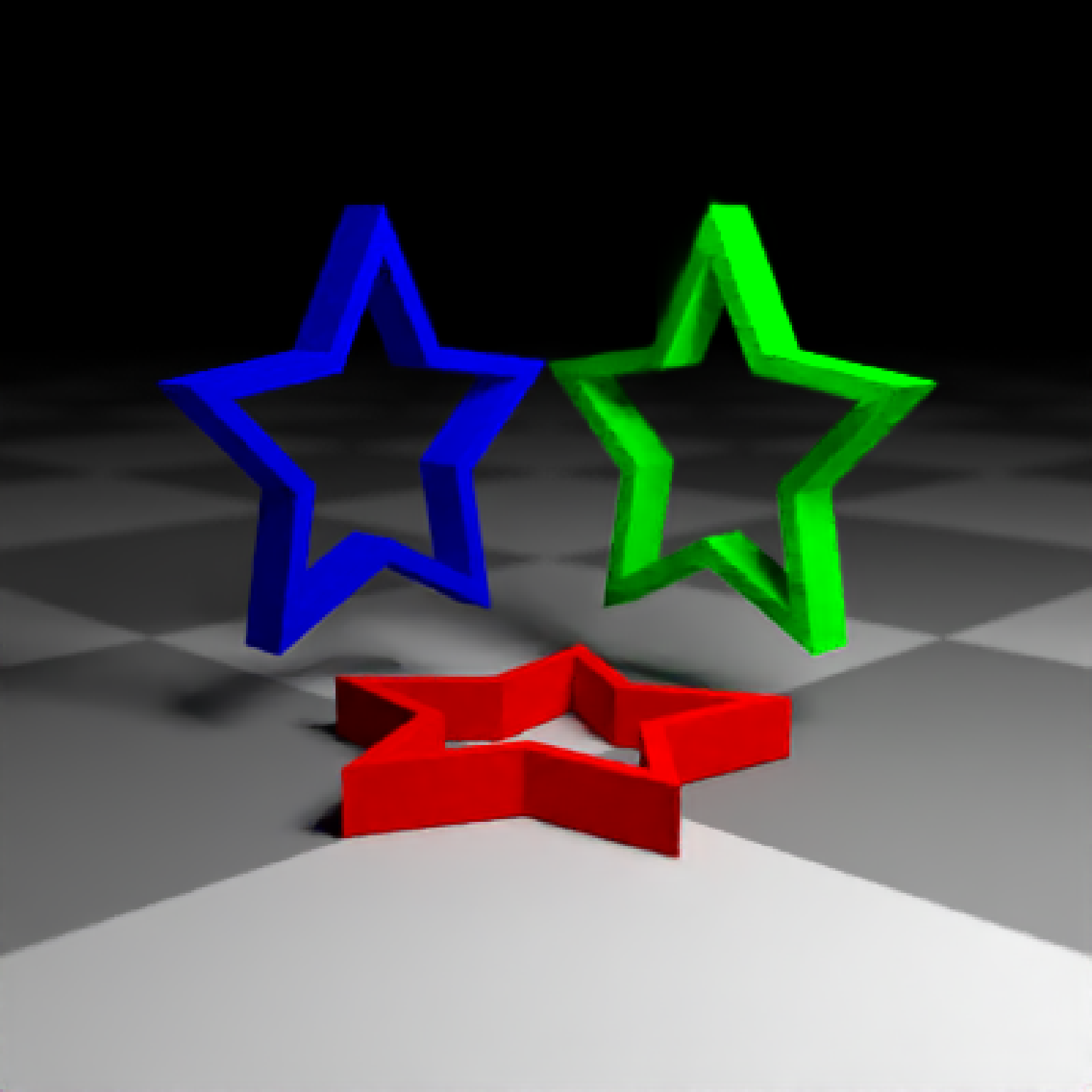#We can also directly pass in sf polygons:
if(run_documentation()) {
if(length(find.package("spData",quiet=TRUE)) > 0) {
us_states = spData::us_states
texas = us_states[us_states$NAME == "Texas",] #Fix no sfc class in us_states geometry data class(texas$geometry) = c("list","sfc")
}
}
#> The legacy packages maptools, rgdal, and rgeos, underpinning the sp package,
#> which was just loaded, will retire in October 2023.
#> Please refer to R-spatial evolution reports for details, especially
#> https://r-spatial.org/r/2023/05/15/evolution4.html.
#> It may be desirable to make the sf package available;
#> package maintainers should consider adding sf to Suggests:.
#> The sp package is now running under evolution status 2
#>      (status 2 uses the sf package in place of rgdal)

#This uses the raw coordinates, unless center = TRUE, which centers the bounding box
#of the polygon at the origin.
if(run_documentation()) {
generate_ground(depth=-0.01,
material = diffuse(color="grey50",checkercolor="grey20")) %>%
material=diffuse(color="#ff2222",sigma=90))) %>%
material=light(color="lightblue",intensity=40))) %>%
render_scene(parallel=TRUE,lookfrom = c(0,10,-10),samples=128,fov=60)
}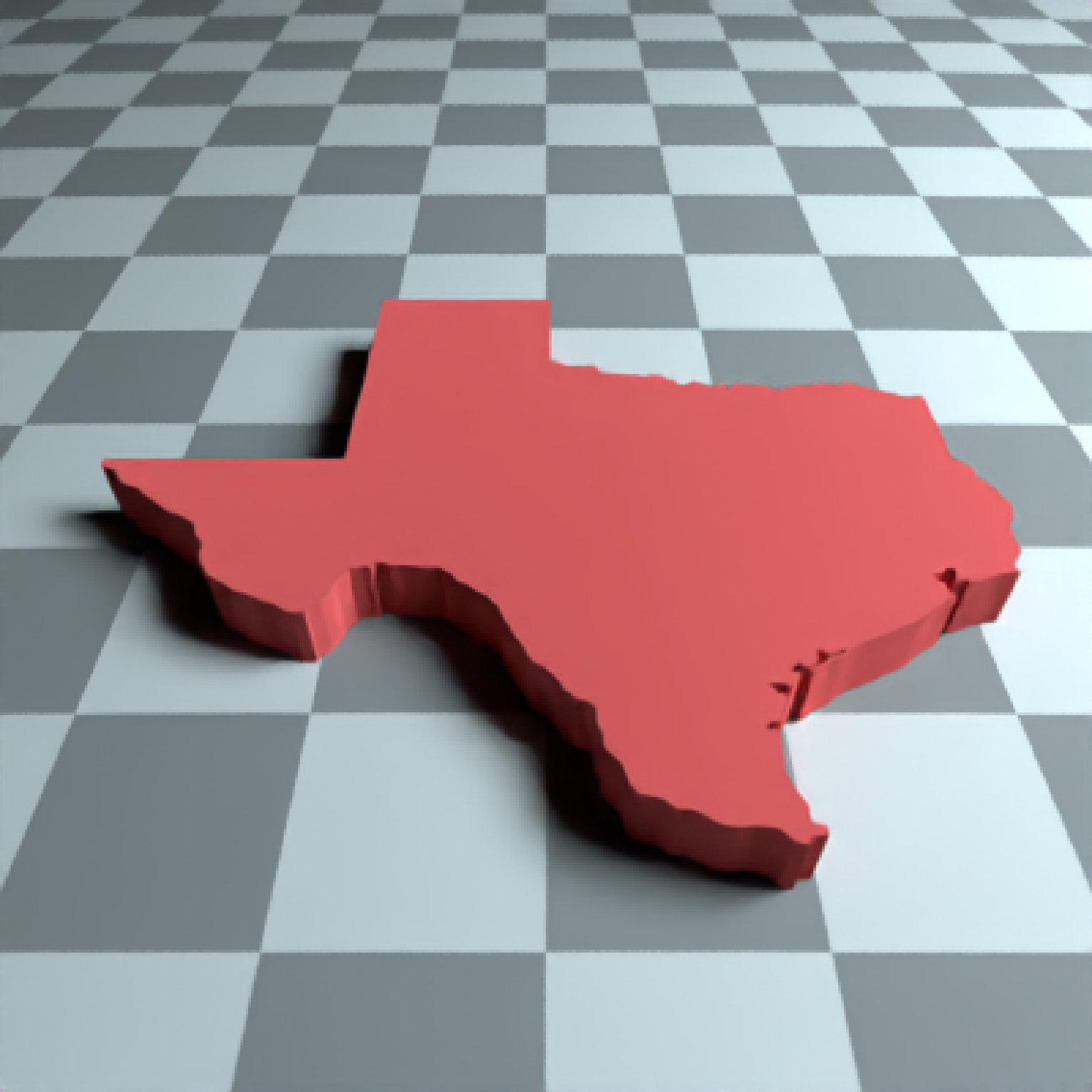#Here we use the raw coordinates, but offset the polygon manually.
if(run_documentation()) {
generate_ground(depth=-0.01,
material = diffuse(color="grey50",checkercolor="grey20")) %>%
material=diffuse(color="#ff2222",sigma=90))) %>%
material=light(color="lightblue",intensity=200))) %>%
material=light(color="orange",intensity=200))) %>%
render_scene(parallel=TRUE,lookfrom = c(0,120,-120),samples=128,fov=20)
}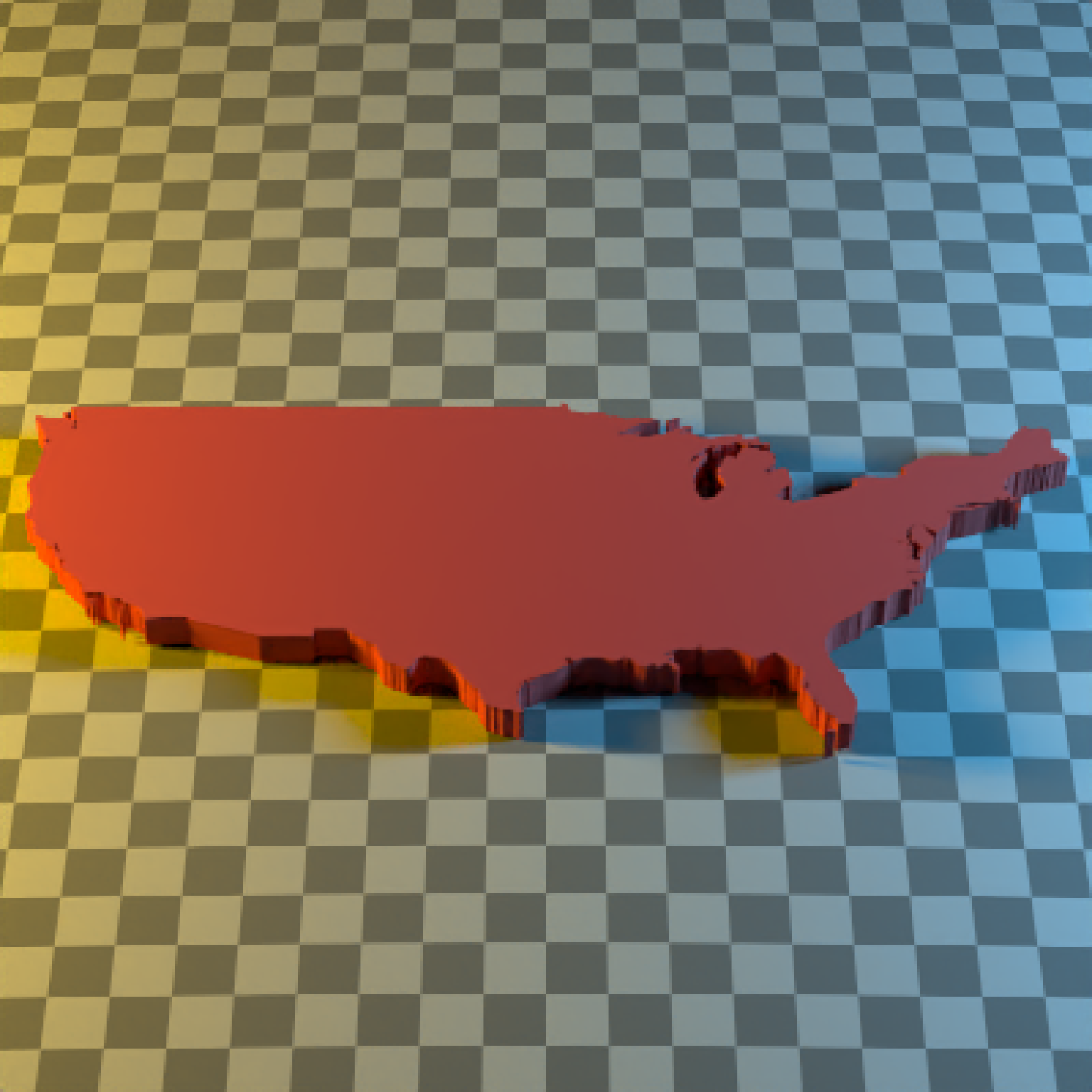#We can also set the map the height of each polygon to a column in the sf object,
#scaling it down by the maximum population state.

if(run_documentation()) {
generate_ground(depth=0,
material = diffuse(color="grey50",checkercolor="grey20",sigma=90)) %>%